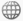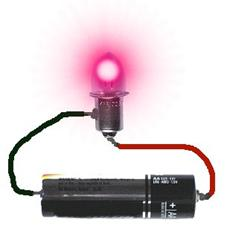# Making sense of voltage

This focus idea is explored through:

## Contrasting student and scientific views

### Student everyday experiencesMost students at this level will have had extensive experience with using electric and electronic devices, both household (such as video and DVD players), and personal (such as iPods and mobile phones). They will often have operational knowledge for using these devices, but it is very unlikely they will have given any thought to the underlying science; their concern is with getting the devices to work but not why they work.

In describing these experiences students use terms such as ‘voltage’, ‘current’, ‘power’ and ‘electricity’, but these are commonly used interchangeably and imprecisely (as noted in the focus ideas Electrostatics – a non-contact force and Electric circuits). Many students at this level will still conceive of a simple electric circuit as having a ‘source’ (called the battery) of some ‘substance’ (called the current) which is ‘consumed’ by whatever the circuit is carrying the ‘substance’ to (such as a light globe, digital camera or iPod).

Many students see combining batteries (e.g. having 4 AA batteries in a device) as giving greater ‘power’. Students tend to think of larger batteries as being somehow more powerful. They accept that for small voltages batteries of different physical sizes can have the same ‘power’ (the common examples are the range of sizes of 1.5V batteries, and the different sizes of 9V batteries in lanterns and smoke alarms).

Sometimes voltage is seen as a property of the electric current: the ‘strength’ of, the ‘intensity’ of, or even the ‘force’ behind the current. In this view, the battery is usually seen as a store of electric current (and/or, more uncommonly, of electric charge), and voltage as a measure of the amount of current in the battery. Often voltage is seen to only exist when there is a current in the circuit/device; sometimes voltage is even seen as caused by current.

A very small number of students are likely to have the idea that voltage is what is ‘used up’ in a circuit (in, for example, a light globe). In this understanding there is voltage ‘before’ the light globe in the circuit, but not ‘after’ it.

There are two very common features of the ways students think about electric circuits, including voltage. The first is that they use local reasoning - they focus their attention on one point in the circuit and ignore what is happening elsewhere. For example, in a circuit with multiple light globes, they believe that if you change something about one light globe nothing else in the circuit would be changed. The second feature is that they use sequential reasoning – they consider circuits as a series of discrete steps that follow one after the other. For example, in a circuit with multiple light globes, they believe that if you change something about one light globe, this will only effect the light globes located ‘after’ this one in the circuit. That is, students very commonly think about electric circuits in terms of single event cause-effect rather than seeing a circuit as an interrelated system where changes to one part affect all other parts.

Research:  Duit (1985), Duit & von Rhöneck (1998), Gunstone, Mulhall & McKittrick (TBP); Maichle (1982), McDermott & van Zee (1985), Millar & King (1993), Reiner Slotta, Chi & Resnick (2000), von Rhöeneck (1985)

### Scientific view

In understanding voltage, it is important to build on the model for electric circuits described in the focus idea Electric Circuits.

In summary, the model has a battery (or other energy source) causing an overall movement of charged particles around a simple circuit. When the circuit is composed of metallic materials, the moving charged particles are electrons. The battery pushes electrons in the circuit away from its negative terminal and pulls them towards the positive terminal (like charges repel, unlike charges attract; see the focus idea Electrostatics – A non-contact force). The energy of the battery to push/pull the electrons comes from chemical reactions occurring within the battery when it is connected in a complete circuit.

The chemical reactions in a battery involve the movement of charged particles inside the battery; this results in an excess of negative charged particles at one battery terminal (the negative terminal) and an excess of positive charged particles at the other terminal. An electron at the negative terminal will be pushed away from this terminal (and pulled towards the positive terminal) if the battery is connected into a circuit.

The excess charges on the battery terminals create pushes and pulls simultaneously on electrons all around the circuit. This pushing and pulling is an example of forces acting at a distance; scientists use the term electric field (see the focus idea Forces without contact) to describe these pushes and pulls. Change at any point in a circuit affects the whole circuit. Because of their position in the electric field electrons in the circuit have electric potential energy. As electrons move in the circuit, the electric potential energy is transformed into low grade heat and whatever useful forms of energy the circuit devices are designed for (see the focus idea Is energy conserved or running out?).

There is a difference in electric potential energy for each charge between any pair of points in a circuit. This is almost always abbreviated to potential difference. An alternative label for potential difference is voltage, a label that comes from our measuring this in volts.

Potential difference and electric field are intertwined. If there is a potential difference in a circuit, then there is an electric field; if there is an electric field in a circuit, then there are forces acting on charged particles in the circuit. Therefore, if there is a voltage in a circuit, then there might be a current (the circuit needs to be complete before there is a current). This form of causality leads many physicists to adopt the simple mantra ‘voltage is the cause, current is the effect’.

Since voltage is the difference in electric potential energy per charge, we should measure it in terms of energy per charge – and we do. ‘Volt’ is an abbreviation for ‘joule per coulomb’, so, for example, a 1.5 volt battery provides 1.5 joule of electric potential energy to each coulomb of charge.

## Critical teaching ideas

• When a battery is connected into a circuit, the excess of positive and negative charges at the battery terminals cause an electric field to exist in the circuit.
• Electrons in the circuit have electric potential energy because of their position in the electric field; there is a difference in electric potential energy (a ‘potential difference’ or a ‘voltage’) between any two points in the circuit.
• Charge gains electric potential energy as it passes through the battery; this is transformed into other energy forms as charge moves around the circuit.
• ‘Voltage’ is about differences in electric potential energy for each change. This is very helpfully reinforced by using ‘joule per coulomb’ instead of ‘volt’ when measuring voltage.
• The greater the voltage, the greater the electric field in a circuit and the greater the electric potential energy per charge transformed as charges move in the circuit.

Research: Gunstone, Mulhall & McKittrick (2009), Loughran, Berry & Mulhall (2006)Explore the relationships between ideas about voltage in the Concept Development Maps – Electricity and Magnetism

Many text books begin any mention of ‘voltage’ with very vague expressions like ‘Voltage can be thought of as …’, ‘Voltage is sometimes described as…’. Other textbooks do not even use the word at all. This is partly because the concept is a very difficult and completely abstract one. In part it is also because understanding the underlying science concepts might not be helped by using ‘voltage’ (it is an imprecise term; for example it is used both in contexts of a difference in electric potential energy between any pair of points and electric potential energy at one point). However it is such a commonly used term that it must be part of teaching of these ideas at this level – concerns with its precision and value are much better left until specialist physics courses.

As with the focus idea Electric circuits, quantitative teaching approaches can impede the development of conceptual understanding. At Level 6 these are still best avoided, or, if they must be introduced, used only after the development of understanding. They certainly should not be the central aspect of assessment of student learning. A teaching focus on mathematical relationships leads to a very strong student learning focus on how concepts are linked from a logical, mathematical viewpoint; it is then very hard for students to explain why concepts are linked this way, what causes those linkages, and, most importantly at this level, why the science is used in the world in the ways it is.Again as detailed in the focus idea Electric circuits, teacher use of language is important here and the use of models, metaphors and analogies is vital in developing students’ understanding. An appropriate model for ‘voltage’ is one related to energy, in particular gravitational potential energy, because the transformation of gravitational potential energy into other forms can be directly observable. An appropriate model is a cliff. An object at the edge of the top of a cliff has gravitational potential energy because of its position in the gravitational field. We could, if we chose, describe the height of the cliff in terms of the gravitational potential energy of the object – a 10 metre high cliff could be described as a 980 joule/kilogram cliff because the difference in gravitational potential energy between the bottom and top of the cliff is 980 joule for each kilogram in any object.

## Teaching activities

### Open discussion via a shared experience and challenge some existing ideasPOE (Predict-Observe-Explain): As a demonstration, show students a simple circuit of a globe lit by a 1.5V, AA size battery.

Now ask students to predict whether the globe will shine more brightly, less brightly or the same when the AA sized battery is replaced by a D size battery (i.e. the original battery is replaced by another battery that is clearly larger in size even though it is the same 1.5 V voltage).

### Practise using and building the perceived usefulness of scientific models

Have students in groups connect up a light globe with a battery so the globe is lit. Then give each group a drawing of the ‘cliff model of gravitational potential energy’ described above.

Ask each group to produce a group response to the following questions:

• What part of the circuit is the drawing of the cliff supposed to be modelling, and why?
• What could be added to the cliff drawing to give a model of the remainder of the circuit?

As a whole class, discuss the responses and draw attention to how the models suggested by the groups are both consistent with and different from the electric circuit. This will further help students with the complex notion that models and analogies always have differences from the observations and concepts they are designed to help understand.Students can also be given a drawing of 2 batteries connected one after the other and asked to describe what a ‘cliff model’ of this arrangement would look like, explaining their response.

Next, ask the students to decide what voltage this arrangement would provide between points A and B in this simple circuit, and why.

### Promote reflection and clarification of existing ideas

Ask students to draw a concept map. This could include terms such as ‘battery’, ‘voltage’, ‘energy’, ‘potential difference’ and ‘electric current’.

Research: Licht (1991), Gunstone, Mulhall & McKittrick (2009), Loughran, Berry & Mu​lhall (2006)